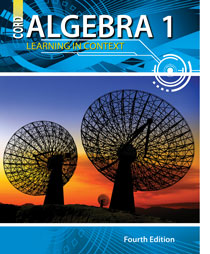# Algebra 1 - 4th Edition

## Chapter 1: Operations, Expressions, and Equations

Some links are repeated for use with more than one lesson.

### 1.1 The Set of Real Numbers

http://mathworld.wolfram.com/RealNumber.html
This page contains a description of the set of real numbers and what it entails.

http://en.wikipedia.org/wiki/Real_number
This article includes a definition of real numbers, their uses and the history behind them.

### 1.2 Absolute Value

http://www.freemathhelp.com/absolute-value.html
This page has notes on the definition of absolute value and how to solve for it.

http://www.coolmath.com/algebra/Algebra1/05AbsValueEq/01_review.htm
This site contains a review on absolute value and shows how to visualize it on a number line.

### 1.3 Adding and Subtracting Real Numbers

http://www.math.com/school/subject1/lessons/S1U1L11DP.html

### 1.5 Variables and Expressions

http://www.freemathhelp.com/Lessons/PreAlgebra_Variables_and_Expressions_BB.htm
This page contains a video tutorial with notes on variables and expressions.

http://www.mathscore.com/math/practice/Single%20Variable%20Equations/
This site has online practice problems with variables.

### 1.6 Equations and Formulas

http://www.mathsisfun.com/algebra/equation-formula.html
This article contains a definition and explanation of equations and formulas with examples.

http://www.math-drills.com/algebra/algebra_missing_numbers_operations_0109_variables_001.html
Printable worksheets with many types of practice problems solving equations. See versions B, C, D, etc.

http://www.coolmath.com/crunchers/algebra-problems-solving-equations-1.htm
http://www.coolmath.com/crunchers/algebra-problems-solving-equations-2.htm
Online practice solving equations with addition and multiplication of variables.